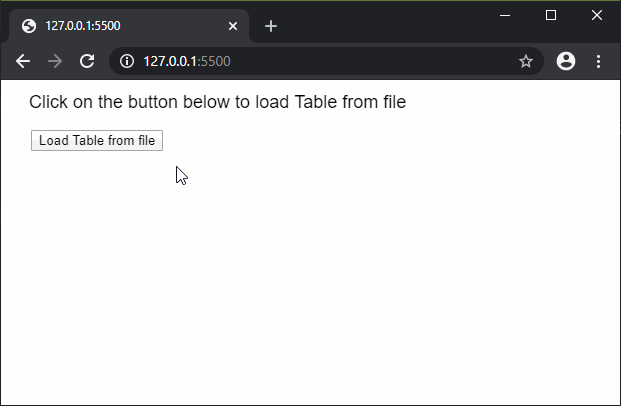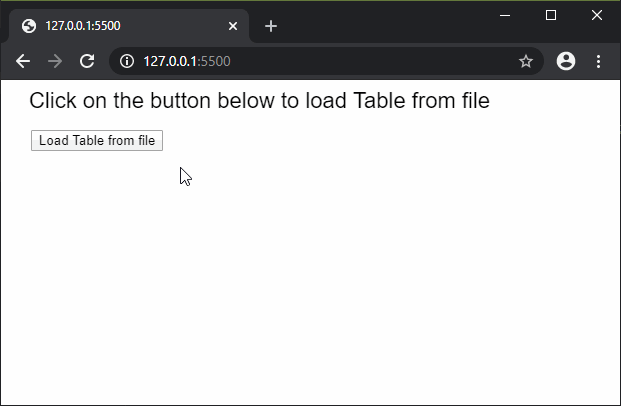# p5.js | loadTable() Function

• Last Updated : 21 Mar, 2020

The loadTable() function is used to read the contents of a file or URL and create a p5.Table object from it. The options parameter can be used to define the type of format that the data is expected to be read. All the files loaded and saved are in UTF-8 encoding.

This function is asynchronous, therefore it is recommended to be called in the preload() function to ensure that the function is executed before the other functions.

Syntax:

`loadTable(filename, options, [callback], [errorCallback])`

or

`loadTable(filename, [callback], [errorCallback])`

Parameters: This function accepts four parameter as mentioned above and described below.

• filename: This is a string which denotes the file path or URL from where the data has to be loaded.
• options: It is a string which denotes the format of the file to be loaded. It can be either “csv” which loads the table using comma-separated-values or “tsv” which loads the table using tab-separated-values. Also the value “header” can be specified to denote if the table has a header value. Multiple commands can be used by passing them as separate parameters. It is an optional parameter.
• callback: This is a function which is called when this function executes successfully. The first argument for this function is the XML data loaded from the file. It is an optional parameter.
• errorCallback: This is a function which is called if there is any error in executing the function. The first argument for this function is the error response. It is an optional parameter.

Below examples illustrate the loadTable() function in p5.js:

Example 1:

 `// Contents of books.csv`` ` `// Book One, Author One, Price One``// Book Two, Author Two, Price Two``// Book Three, Author Three, Price Three`` ` `let loadedTable = ``null``;`` ` `function` `setup() {``  ``createCanvas(500, 300);``  ``textSize(18);`` ` `  ``text(``"Click on the button below to"``+``       ``" load Table from file"``, 20, 20);`` ` `  ``// Create a button for loading the table``  ``loadBtn = createButton(``"Load Table from file"``);``  ``loadBtn.position(30, 50)``  ``loadBtn.mousePressed(loadFile);``}`` ` `function` `loadFile() {`` ` `  ``// Load the table from file``  ``loadedTable = loadTable(``'books.csv'``, ``'csv'``, onFileload);``}`` ` `function` `onFileload() {``  ``text(``"Table loaded successfully..."``, 20, 100);`` ` `  ``// Display through the table``  ``for` `(let r = 0; r < loadedTable.getRowCount(); r++) {``    ``for` `(let c = 0; c < loadedTable.getColumnCount(); c++) {``      ``text(loadedTable.getString(r, c), ``            ``20 + c * 200, 150 + r * 20);``    ``}``  ``}``}`

Output:Example 2:

 `// Contents of books_header.csv`` ` `// title, author, price``// Book One, Author One, Price One``// Book Two, Author Two, Price Two``// Book Three, Author Three, Price Three`` ` `let loadedTable = ``null``;`` ` `function` `setup() {``  ``createCanvas(500, 300);``  ``textSize(22);`` ` `  ``text(``"Click on the button below to "``       ``+ ``"load Table from file"``, 20, 20);`` ` `  ``// Create a button for loading the table``  ``loadBtn = createButton(``"Load Table from file"``);``  ``loadBtn.position(30, 50)``  ``loadBtn.mousePressed(loadFile);``}`` ` `function` `loadFile() {``  ``// Load the table from file with headers``  ``loadedTable = loadTable(``'books_header.csv'``,``                ``'csv'``, ``'header'``, onFileload);``}`` ` `function` `onFileload() {``  ``text(``"Table loaded successfully..."``, 20, 100);`` ` `  ``// Display the headers``  ``for` `(let h = 0; h < loadedTable.getColumnCount(); h++) {``    ``text(loadedTable.columns[h], 20 + h * 200, 150);``  ``}`` ` `  ``textSize(16);``  ``// Display the data in the table``  ``for` `(let r = 0; r < loadedTable.getRowCount(); r++) {``    ``for` `(let c = 0; c < loadedTable.getColumnCount(); c++) {``      ``text(loadedTable.getString(r, c), ``            ``20 + c * 200, 170 + r * 20);``    ``}``  ``}``}`

Output:Online editor: https://editor.p5js.org/

My Personal Notes arrow_drop_up